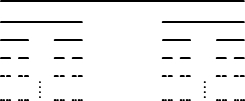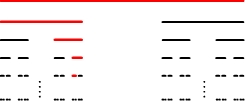# How Many Elements Are There in the Cantor Set?

##### Age 14 to 18

Published 2007 Revised 2017

What is the Cantor set?

Consider the unit interval [0,1]. Remove the middle third of this interval, (1/3,2/3), so that you are left with two pieces, [0,1/3] and [2/3,1]. Next remove the middle third from each of these two intervals. That is, remove (1/9,2/9) from the first interval, and remove (7/9,8/9) from the second interval. We are now left with four pieces: [0,1/9], [2/9,3/9], [6/9,7/9], and [8/9,1]. Repeat the procedure again and remove the middle third from each of these four intervals, so that we are left with eight pieces. Continue this process indefinitely. Those points in [0,1] that do not eventually get removed in the procedure constitute a set, which is called the Cantor set . An illustration of the construction of the Cantor set is shown below. The top line is the unit interval [0,1]. The second line consists of [0,1] with its middle third removed, and so forth.Is there anything left after removing all these middle thirds? Certainly; for example, the Cantor set contains the points 0, 1/3, 25/27, and 1. The purpose of this article is to discuss exactly how many points there are in the Cantor set.

How many points are there in the Cantor set?

Each point of the Cantor set lies at the root of an infinite nested sequence of intervals. For example, consider the diagram below.One interval from each step has been coloured in red. Each red interval, other than the top one, lies below another red interval. This nested sequence of red intervals shrinks down to a single point. This single point is contained in every one of the red intervals. It is not deleted in the infinite process of removing middle thirds of intervals described in the previous section, so it is a member of the Cantor set. In fact, we can describe each pointof the Cantor set in this fashion; to each point in the Cantor set there corresponds a unique infinite sequence of nested (red) intervals.

We need a way to label a point in the Cantor set according to the path of red intervals that is taken to reach it. Let us label each point by an infinite sequence consisting of 0s and 1s. The first entry in the sequence is 0 if the point lies in the left hand interval after the first stage of the Cantor process, and 1 if it lies in the right hand interval. In the second stage of the Cantor process, the interval in which the point was found to lie after the first stage gets split into two more intervals: a left interval and a right interval. We label the second entry in our sequence 0 if the point lies in the left interval, and 1 if it lies in the right interval. This process is repeated indefinitely. For example, in the diagram above, the point contained in all the red intervals is represented by the sequence 0110.... (the dots indicate that the sequence of 0s and 1s is infinite).The point 0 in [0,1] is represented by the sequence 0000.... and the point 1 is represented by the sequence 1111.... The point 1/3 is represented by the sequence 01111....

We now have a way of understanding how many points there are in the Cantor set. There are as many points in the Cantor set as there are infinite sequences consisting of 0s and 1s. If we place a point before any one of these infinite sequences--for example, "0100010...." becomes ".0100010..."--then we convert an infinite sequence of 0s and 1s to the binary expansion of a real number between 0 and 1. This means that the number of points in the Cantor set is the same as the number of points in the interval [0,1]. We conclude that the infinite process of removing middle thirds from the interval [0,1] has no effect on the number of points in [0,1] after all!

For an alternative description of the size of the Cantor set, see the Notes on The Cantor Set. You might like to try the problems: The Cantor Set and How Long is The Cantor Set? For more information on the number of points in [0,1], see Infinity is not a Number - It's a Free Man .